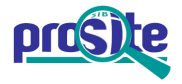Entry: PS00266

 Entry name [info] SOMATOTROPIN_1 Accession [info] PS00266 Entry type [info] PATTERN Date [info] 01-APR-1990 CREATED; 01-DEC-2004 DATA UPDATE; 02-JUN-2021 INFO UPDATE. PROSITE Doc. [info] PDOC00239

Name and characterization of the entry

 Description [info] Somatotropin, prolactin and related hormones signature 1. Pattern [info] ```C-x-[STN]-x(2)-[LIVMFYS]-x-[LIVMSTA]-P-x(5)-[TALIV]-x(7)-[LIVMFY]-x(6)- [LIVMFY]-x(2)-[STACV]-W. ```

Numerical results [info]

Numerical results for UniProtKB/Swiss-Prot release 2021_03 which contains 565'254 sequence entries.

 Total number of hits 197 in 197 different sequences Number of true positive hits 191 in 191 different sequences Number of 'unknown' hits 0 Number of false positive hits 6 in 6 different sequences Number of false negative sequences 25 Number of 'partial' sequences 2 Precision (true positives / (true positives + false positives)) 96.95 % Recall (true positives / (true positives + false negatives)) 88.43 %

 UniProtKB/Swiss-ProtTrue positive sequences 191 sequences ```CSH1_BOVIN (P09611 ), CSH1_HUMAN (P0DML2 ), CSH2_BOVIN (P19159 ), ``` ```CSH2_HUMAN (P0DML3 ), CSH_SHEEP (P16038 ), PLRP1_BOVIN (P05402 ), PLRP2_BOVIN (P12401 ), PLRP3_BOVIN (P12402 ), PR2A1_MOUSE (Q9JHK0 ), PR2C2_MOUSE (P04095 ), PR2C3_MOUSE (P04768 ), PR2C5_MOUSE (Q9JLV9 ), PR3B1_MESAU (P14059 ), PR3B1_MOUSE (P09586 ), PR3B1_RAT (P09321 ), PR3D1_MOUSE (P18121 ), PR3D1_RAT (P21702 ), PR3D4_RAT (P34207 ), PR4A1_MOUSE (O35256 ), PR4A1_RAT (P09320 ), PR7B1_MOUSE (Q8CGZ9 ), PR7B1_RAT (Q8CJ42 ), PR7C1_MOUSE (Q9CRB5 ), PR7D1_MOUSE (P04769 ), PRL1_ALLMI (P55751 ), PRL1_CRONO (P55753 ), PRL1_ONCKE (P69130 ), PRL1_ONCTS (P69131 ), PRL1_OREMO (P09319 ), PRL2_ALLMI (P55752 ), PRL2_CRONO (P55754 ), PRL2_ONCKE (P09584 ), PRL2_ONCTS (Q91364 ), PRL2_OREMO (P09318 ), PRL_AILME (Q8HXS1 ), PRL_ANGAN (P33096 ), PRL_ANGJA (Q7ZZV3 ), PRL_BALBO (P33089 ), PRL_BOVIN (P01239 ), PRL_CAMDR (P22393 ), PRL_CAPHI (Q28318 ), PRL_CARAU (P87495 ), PRL_CEREL (Q6UC74 ), PRL_CHEMY (P33090 ), PRL_CHICK (P14676 ), PRL_CORAU (P34181 ), PRL_CYPCA (P09585 ), PRL_DICLA (P48249 ), PRL_FELCA (P46403 ), PRL_HORSE (P12420 ), PRL_HUMAN (P01236 ), PRL_HYPMO (P35395 ), PRL_HYPNO (P29235 ), PRL_ICTPU (P51904 ), PRL_ISOMA (Q3Y4G6 ), PRL_LOXAF (P10765 ), PRL_MACMU (P55151 ), PRL_MELGA (P17572 ), PRL_MESAU (P37884 ), PRL_MICMO (Q9QZL1 ), PRL_MONDO (O62819 ), PRL_NEOVI (P29234 ), PRL_ONCMY (P21993 ), PRL_PAROL (Q9YGV6 ), PRL_PIG (P01238 ), PRL_PROAT (P33091 ), PRL_RABIT (Q28632 ), PRL_RAT (P01237 ), PRL_SALSA (P48096 ), PRL_SHEEP (P01240 ), PRL_SPAAU (O93337 ), PRL_TRIVU (O62781 ), SOM2_HUMAN (P01242 ), SOM2_MACMU (Q07370 ), SOM2_PANTR (P58757 ), SOMA1_ACIGU (P26773 ), SOMA1_CARAU (O93359 ), SOMA1_ONCMY (P09538 ), SOMA1_ONCNE (Q91222 ), SOMA2_ACIGU (P26774 ), SOMA2_CARAU (O93360 ), SOMA2_ONCMY (P20332 ), SOMA2_ONCNE (Q91221 ), SOMA2_XENLA (P12856 ), SOMA_ACABU (Q01282 ), SOMA_ACALA (P45654 ), SOMA_AILME (Q8HYE5 ), SOMA_ANAPL (P11228 ), SOMA_ANGJA (P08899 ), SOMA_BALBO (P33092 ), SOMA_BALPH (Q659Q8 ), SOMA_BOSGF (Q1HFN3 ), SOMA_BOSMU (Q864S7 ), SOMA_BOVIN (P01246 ), SOMA_BUBBU (O18938 ), SOMA_CALJA (Q9GMB3 ), SOMA_CAMDR (Q7YRR6 ), SOMA_CANLF (P33711 ), SOMA_CAPHI (P67931 ), SOMA_CAVPO (Q9JKM4 ), SOMA_CEREL (P56437 ), SOMA_CHEMY (P34005 ), SOMA_CHICK (P08998 ), SOMA_CORAU (P45655 ), SOMA_CORLV (O13188 ), SOMA_CRONO (P55755 ), SOMA_CTEID (P69158 ), SOMA_CYPCA (P10298 ), SOMA_DELDE (Q8MI73 ), SOMA_DICLA (Q05163 ), SOMA_ESOLU (P34744 ), SOMA_FELCA (P46404 ), SOMA_GALSE (Q9GKA1 ), SOMA_GIRCA (Q7YQD2 ), SOMA_HETFO (Q9W6R8 ), SOMA_HIPAM (Q7YQB8 ), SOMA_HORSE (P01245 ), SOMA_HUMAN (P01241 ), SOMA_HYPMO (P69159 ), SOMA_HYPNO (P69160 ), SOMA_ICTPU (P34745 ), SOMA_KATPE (P20391 ), SOMA_LABRO (Q9W6J7 ), SOMA_LARCR (Q9I9M4 ), SOMA_LATCA (Q01283 ), SOMA_LEPOS (P79885 ), SOMA_LITCT (P10813 ), SOMA_LOXAF (P20392 ), SOMA_MACMU (P33093 ), SOMA_MELGA (P22077 ), SOMA_MESAU (P37886 ), SOMA_MISMI (Q9W6J5 ), SOMA_MONDO (Q9GL60 ), SOMA_MORSA (P48248 ), SOMA_MOUSE (P06880 ), SOMA_NEOVI (P19795 ), SOMA_NYCPY (Q9GMB2 ), SOMA_ODOAR (Q9I9L5 ), SOMA_ONCKE (P07064 ), SOMA_ONCKI (P10607 ), SOMA_ONCMA (Q9DGG5 ), SOMA_ONCTS (Q07221 ), SOMA_OREMO (P34746 ), SOMA_ORENI (P13391 ), SOMA_PAGMA (P08591 ), SOMA_PANGG (P69162 ), SOMA_PANPG (P69161 ), SOMA_PANTR (P58756 ), SOMA_PAROL (P09537 ), SOMA_PERFV (Q9DEV3 ), SOMA_PIG (P01248 ), SOMA_PRIGL (P34006 ), SOMA_PROAN (O73848 ), SOMA_PSEDN (P24363 ), SOMA_RABIT (P46407 ), SOMA_RAT (P01244 ), SOMA_RHIMB (O73849 ), SOMA_SAIBB (P58343 ), SOMA_SALSA (P10814 ), SOMA_SCIOC (Q9IB11 ), SOMA_SEBSC (P87391 ), SOMA_SERQU (P09539 ), SOMA_SHEEP (P67930 ), SOMA_SIGGU (Q9IBE5 ), SOMA_SOLSE (P45643 ), SOMA_SPAAU (P29971 ), SOMA_SPAEH (O70615 ), SOMA_STRCA (Q9PWG3 ), SOMA_TAKRU (O12980 ), SOMA_THUAL (P34747 ), SOMA_THUTH (P09113 ), SOMA_TRITC (Q98UF6 ), SOMA_TRIVU (O62754 ), SOMA_VERVA (O93566 ), SOMA_VICPA (P37885 ), SOMA_VULVU (P10766 ), SOML1_SPAAU (P54863 ), SOML2_SPAAU (P79894 ), SOML_ACITR (O93262 ), SOML_CYCLU (P45640 ), SOML_GADMO (P21919 ), SOML_HIPHI (P45641 ), SOML_ICTPU (Q9I8J9 ), SOML_ONCKE (P24405 ), SOML_ORYLA (Q6E211 ), SOML_PAROL (P20362 ), SOML_PROAN (O73847 ), SOML_SCIOC (Q9YGK7 ), SOML_SIGGU (Q9PWG4 ), SOML_SOLSE (P45642 ), SOML_TETMU (Q9I9H4 ) ``` » more Retrieve an alignment of UniProtKB/Swiss-Prot true positive hits: [Clustal format, color, condensed view] [Clustal format, color] [Clustal format, plain text] [Fasta format] Retrieve the sequence logo from the alignment UniProtKB/Swiss-ProtFalse negative sequences 25 sequences ```CSHL_HUMAN (Q14406 ), PLRP4_BOVIN (P18917 ), PR2A1_RAT (Q9JII3 ), ``` ```PR2B1_MOUSE (Q9DAZ2 ), PR2B1_RAT (Q9JKL9 ), PR3C1_MOUSE (Q9QUN5 ), PR3C1_RAT (Q9QUL0 ), PR5A1_MOUSE (Q9JII2 ), PR5A1_RAT (Q9JII4 ), PR6A1_MOUSE (O35257 ), PR6A1_RAT (P24800 ), PR7A1_MOUSE (O54830 ), PR7A2_MOUSE (O54831 ), PR7A2_RAT (Q9R006 ), PR8A4_RAT (P33580 ), PR8A5_RAT (P33579 ), PR8A6_MOUSE (Q9DAY2 ), PR8A7_RAT (P33578 ), PR8A8_MOUSE (Q9DAS4 ), PR8A9_MOUSE (Q9CQ58 ), PR8A9_RAT (Q920I0 ), PRL_MOUSE (P06879 ), SOMA1_XENLA (P12855 ), SOML_ANGAN (Q90216 ), SOML_CARAU (P79697 ) ``` » more UniProtKB/Swiss-Prot'Partial' sequences 2 sequences ```GHR4_RAT (P33581 ), PRL_BUFJA (P43001 ) ``` UniProtKB/Swiss-ProtFalse positive sequences 6 sequences ```GD_HHV11 (Q69091 ), GD_HHV1A (P36318 ), GD_HHV1F (Q05059 ), ``` ```GD_HHV1H (P06476 ), GD_HHV1K (A1Z0Q5 ), GD_HHV1P (P57083 ) ``` » more PDB[Detailed view] 24 PDB 1A22; 1AXI; 1BP3; 1F6F; 1HGU; 1HUW; 1HWG; 1HWH; 1KF9; 1RW5; 1Z7C; 2Q98; » more